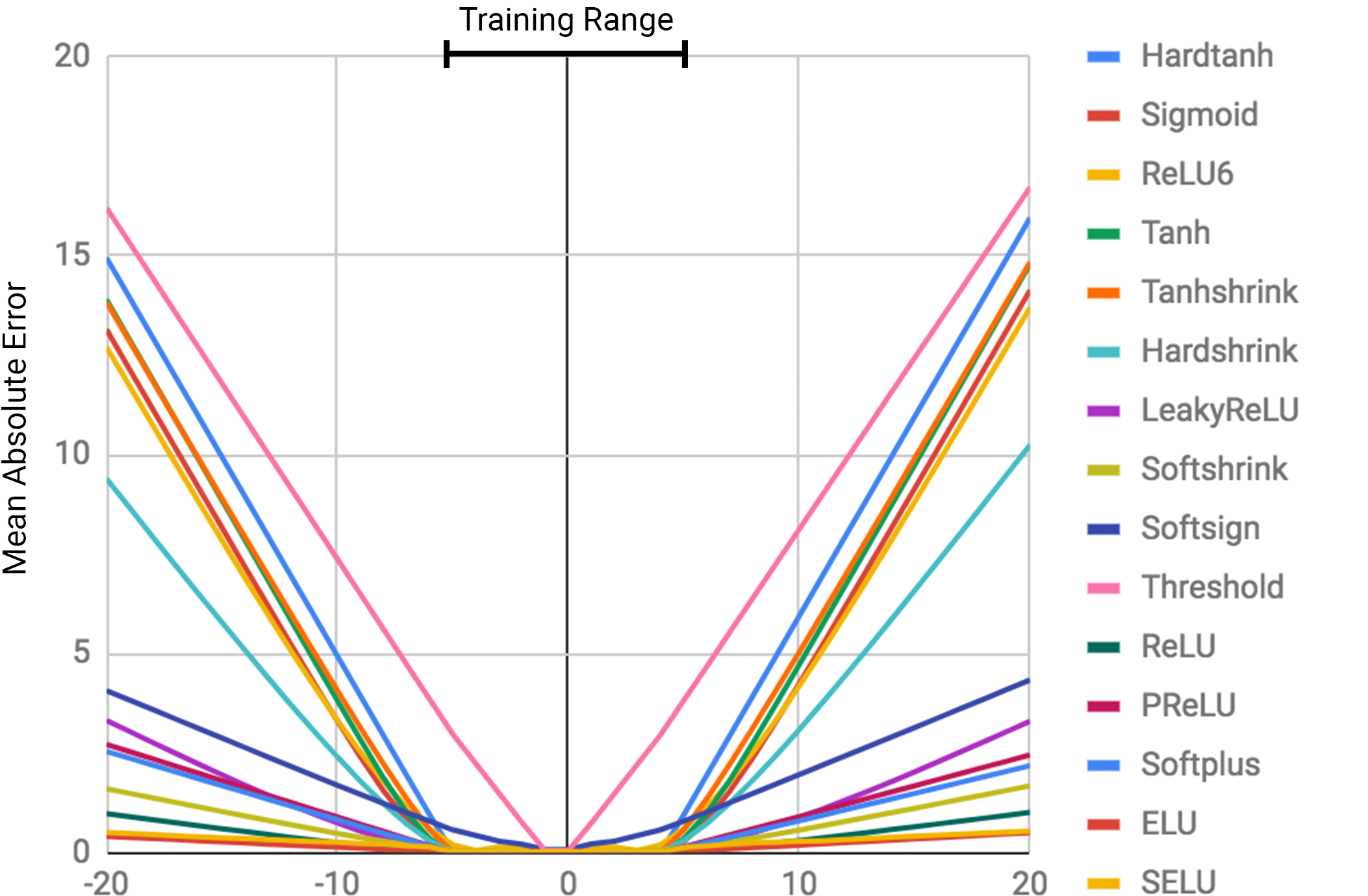# Neural Arithmetic Logic Units

(Trask et al., 2018) on arXiv

It’s time to restart in preparation for the new semester!

Neural networks have trouble with things as simple to us as learning the identity function $f(x) = x$. Within the numerical range they’re trained on, they do fine, but they can’t extrapolate.The paper proposes two new models that generalize better. The first, for better addition and subtraction, is the neural accumulator (NAC). It’s like a normal linear layer, but there’s no bias, and its weight matrix $\mathbf{W}$ is only 0’s, 1’s, and −1’s. Well, sort of. Hard constraints are not differentiable. Instead, they express $\mathbf{W} = \tanh(\mathbf{\hat{W}}) \odot \sigma(\mathbf{\hat{M}})$. The tanh values range from -1 to 1, the sigmoid ranges from 0 to 1, and the values of both are strongly encouraged toward the extremes, so each element-wise product is pushed to the values we want.

The second is a neural arithmetic logic unit (NALU), christened after the structures you remember from your computer architecture classes. It combines two of these NACs, one of which operates in log-space, so it can perform multiplication and division. ($\exp(\log(a) + \log(b)) \equiv a \times b$.) A gate controls whether the NALU takes the add/subtract route or the multiply/divide route.

Their results show that normal neural architectures do poorly at generalizing, even if they can handle the simple addition and subtraction. The NAC does just as well, but the NALU is the only variant that learns to extrapolate beyond the range of training values. When the values are fed in sequentially (rather than as one vector), the LSTM and ReLU-activated RNN both learn interpolation, but fail at extrapolation. NALU learns most extrapolation, but it fails miserably on division. (They justify this by the fact that small denominators can tease out the error in generalization ability.)

They also test performance on two other tasks: MNIST digit counting and digit adding. Both involve recognizing images of digits, then using them. (Digit counting involves a swarm of numbers in each picture.) The NAC (designed for addition and subtraction) wins at this. It’s easily incorporated into existing image recognition architectures by replacing the final layer.

Another task is interpreting text representations of numbers, turning them into text. Here, the NALU shines.

The takeaway:

• We have a single ‘neuron’ that can better handle math.
• It’s easily integrable into existing image classification networks or text interpretation.
Written on August 8, 2018